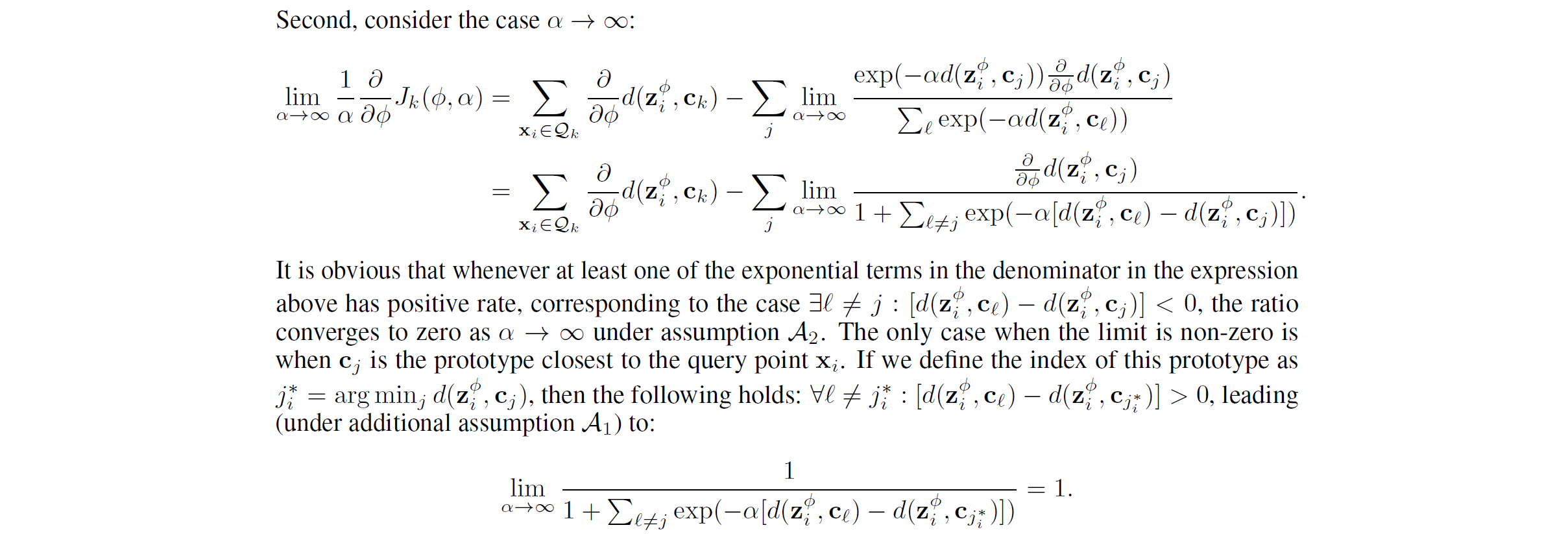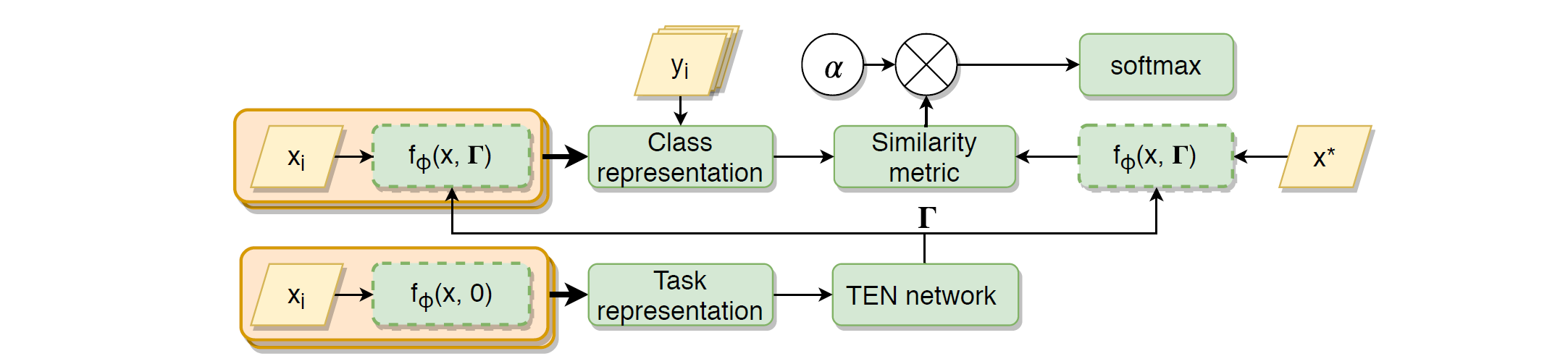Jarvis' Blog (总有美丽的风景让人流连) 总有美丽的风景让人流连

# 少样本学习中的度量函数 (Metrics in FSL)

2020-07-09
Jarvis
Post

### 1.1 Metric Scaling

$p_{\phi}(y=k\vert x) = \text{softmax}(-d(\mathbf{z}, \mathbf{c}_k)),$

$p_{\phi, \alpha}(y=k\vert x) = \text{softmax}(-\alpha d(\mathbf{z}, \mathbf{c}_k)),$

$J_k(\phi, \alpha) = \sum_{x_i\in \mathcal{Q}_k,} \left[ \alpha d(\mathbf{z}, \mathbf{c}_k) + \log\sum_j\exp(-\alpha d(\mathbf{z}, \mathbf{c}_j)) \right], \quad z=f_{\phi}(x_i),$

$\frac{\partial}{\partial\phi}J_k(\phi, \alpha) = \alpha\sum_{x_i\in \mathcal{Q}_k,}\left[ \frac{\partial}{\partial\phi}d(\mathbf{z}, \mathbf{c}_k) - \frac{\sum_j\exp(-\alpha d(\mathbf{z}, \mathbf{c}_j))\frac{\partial}{\partial\phi}d(\mathbf{z}, \mathbf{c}_j)}{\sum_j\exp(-\alpha d(\mathbf{z}, \mathbf{c}_j))} \right].$

$\mathcal{A}_1:\; d(f_{\phi}(x), \mathbf{c}_k) \neq d(f_{\phi}(x'), \mathbf{c}_k), \forall k, x\neq x'\in \mathcal{Q}_k,; \quad \mathcal{A}_2:\; \left\vert \frac{\partial}{\partial\phi}d(f_{\phi}(x), c) \right\vert<\infty, \forall x, c, \phi,$

\begin{align} \lim_{\alpha\rightarrow0}\frac1{\alpha}\frac{\partial}{\partial\phi}J_k(\phi, \alpha) &= \sum_{x_i\in \mathcal{Q}_k,} \left[ \frac{K-1}{K}\frac{\partial}{\partial\phi}d(\mathbf{z}, \mathbf{c}_k) - \frac1K\sum_{j\neq k}\frac{\partial}{\partial\phi}d(\mathbf{z}, \mathbf{c}_j) \right], \\ \lim_{\alpha\rightarrow\infty}\frac1{\alpha}\frac{\partial}{\partial\phi}J_k(\phi, \alpha) &= \sum_{x_i\in \mathcal{Q}_k,} \left[ \frac{\partial}{\partial\phi}d(\mathbf{z}, \mathbf{c}_k) - \frac{\partial}{\partial\phi}d(\mathbf{z}, c_{j^*_i}) \right]; \end{align}• 当 $$\alpha$$ 很小时, 第一个式子右侧第一项是使得极小化了查询样本 $$z$$ 和对应类别的原型 $$\mathbf{c}_k$$ 之间的距离, 第二项极大化了 $$z$$ 和不同类别原型之间的距离.

• 当 $$\alpha$$ 很大时, 第二个式子右侧第一项一样, 第二项极大化 $$z$$ 和离 $$z$$ 最近的不同类别原型之间的距离 (有点 maxmin 的感觉). 如果 $$j^*_i=k$$, 则第二个式子值为 $$0$$. 这表明在 $$\alpha$$ 很大时, 模型主要从困难样本中学习 (maxmin 的体现).